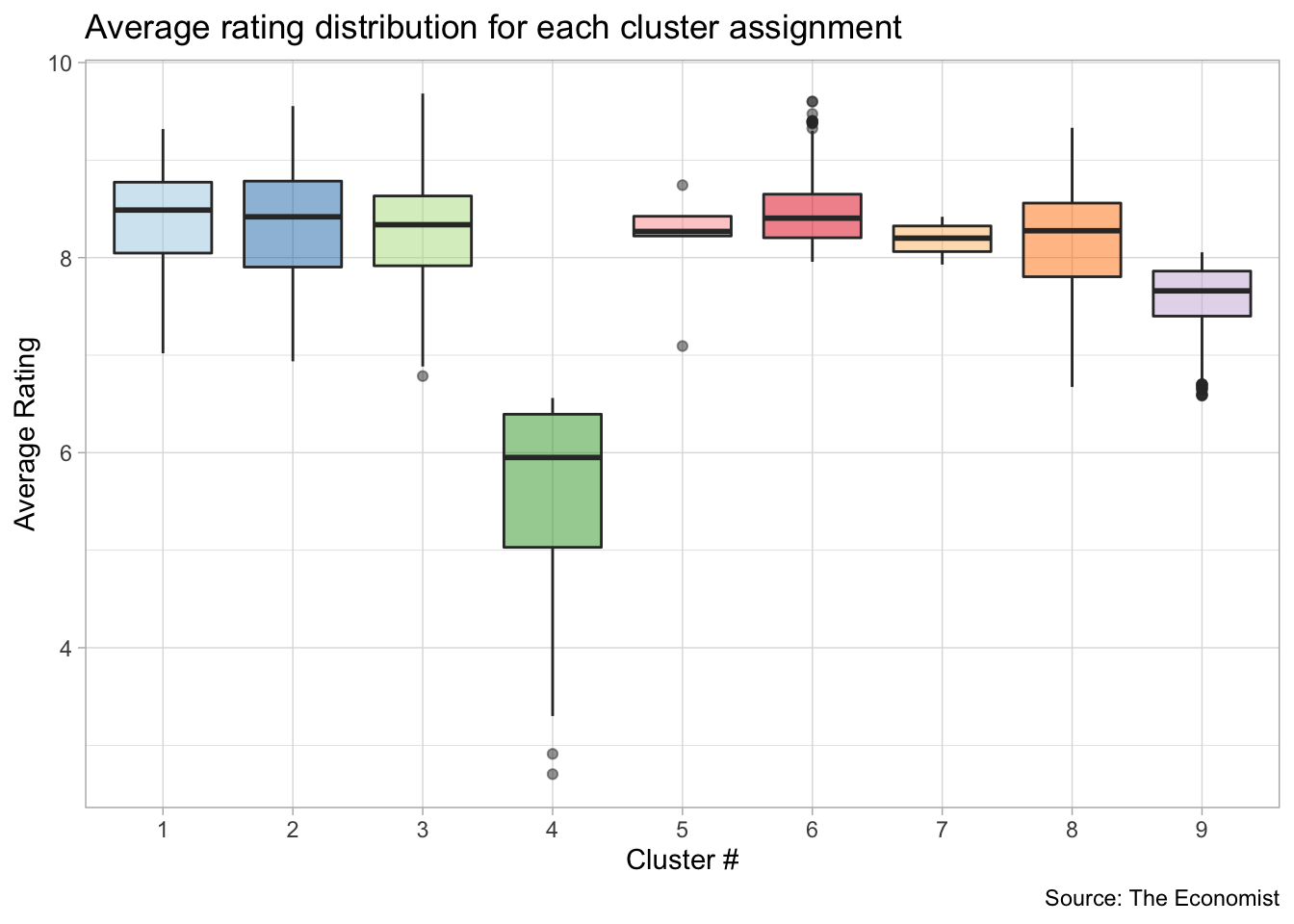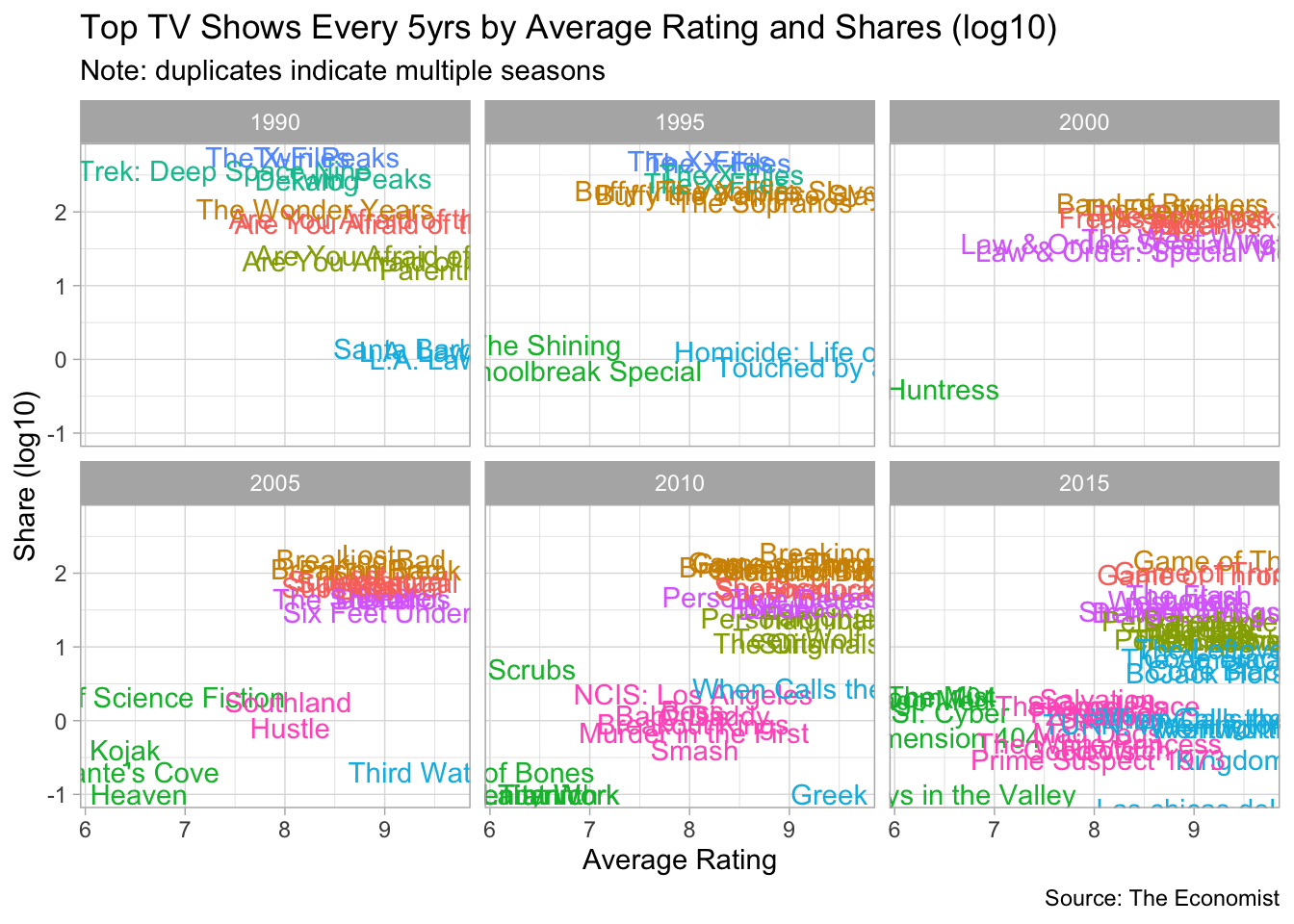Analyzing data for #tidytuesday week of 01/08/2019 (source)

``````# LOAD PACKAGES AND PARSE DATA
library(knitr)
library(tidyverse)
library(RColorBrewer)
library(forcats)
library(lubridate)
library(broom)

tv_data <- tv_data_raw``````

### Prepare the data for k-means clustering

``````tv_data_summarized <- tv_data %>%
group_by(title, genres, date) %>%
summarize(min_rating = min(av_rating),
avg_rating = mean(av_rating),
max_rating = max(av_rating),
min_share = min(share),
avg_share = mean(share),
max_share = max(share)) %>%
ungroup()

kclust_data <- tv_data_summarized %>%
select(-title, -genres, -date)

kclust_results <- kmeans(kclust_data, center = 9)``````

### Check output data (boxplot)

``````# CHECK OUTPUT DATA
tv_data_summarized %>%
left_join(augment(kclust_results, kclust_data)) %>%
mutate(title = factor(title)) %>%
group_by(.cluster) %>%
ggplot() +
geom_boxplot(aes(.cluster, avg_rating, fill = .cluster),
show.legend = FALSE,
alpha = 0.5) +
theme_light() +
labs(x = "Cluster #",
y = "Average Rating",
caption = "Source: The Economist",
title = "Average rating distribution for each cluster assignment") +
scale_fill_brewer(palette = 'Paired')``````### Check outputdata (scatterplot)

``````tv_data_summarized %>%
left_join(augment(kclust_results, kclust_data)) %>%
mutate(title = factor(title)) %>%
group_by(.cluster) %>%
ggplot(aes(avg_rating, log10(avg_share)+1, color = .cluster)) +
geom_point(alpha = 0.7, size = 3, show.legend = FALSE) +
theme_light() +
labs(x = "Average Rating",
y = "Share (log10)",
caption = "Source: The Economist",
title = "Relationship between Average Rating and Shares by cluster assignment") +
scale_fill_brewer(palette = 'Paired')``````### Finalize the plot

``````tv_data_summarized %>%
left_join(augment(kclust_results, kclust_data)) %>%
mutate(title = factor(title),
five_years = 5 * (year(date) %/% 5 )) %>%
group_by(.cluster) %>%
top_n(20, avg_rating) %>%
ggplot(aes(avg_rating, log10(avg_share)+1, label = title, color = .cluster)) +
geom_text(show.legend = FALSE) +
facet_wrap(~five_years) +
theme_light() +
labs(x = "Average Rating",
y = "Share (log10)",
caption = "Source: The Economist",
title = "Top TV Shows Every 5yrs by Average Rating and Shares (log10)",
subtitle = "Note: duplicates indicate multiple seasons") ``````